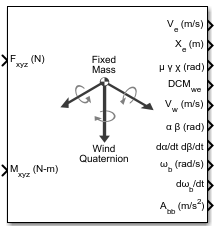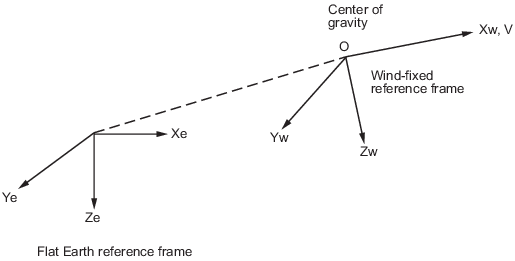# 6DOF Wind (Quaternion)

Implement quaternion representation of six-degrees-of-freedom equations of motion with respect to wind axes

• Library:
• Aerospace Blockset / Equations of Motion / 6DOF

•## Description

The 6DOF Wind (Quaternion) block considers the rotation of a wind-fixed coordinate frame (Xw, Yw, Zw) about an flat Earth reference frame (Xe, Ye, Ze). For more information on the wind-fixed coordinate frame, see Algorithms.

Aerospace Blockset™ uses quaternions that are defined using the scalar-first convention.

## Limitations

The block assumes that the applied forces act at the center of gravity of the body, and that the mass and inertia are constant.

## Ports

### Input

expand all

Applied forces, specified as a three-element vector.

Data Types: `double`

Applied moments, specified as a three-element vector.

Data Types: `double`

### Output

expand all

Velocity in the flat Earth reference frame, returned as a three-element vector.

Data Types: `double`

Position in the flat Earth reference frame, returned as a three-element vector.

Data Types: `double`

Wind rotation angles [bank, flight path, heading], returned as a three-element vector, in radians.

Data Types: `double`

Coordinate transformation from flat Earth axes to wind-fixed axes, returned as a 3-by-3 matrix.

Data Types: `double`

Velocity in wind-fixed frame, returned as a three-element vector.

Data Types: `double`

Angle of attack and sideslip angle, returned as a two-element vector, in radians.

Data Types: `double`

Rate of change of angle of attack and rate of change of sideslip angle, returned as a two-element vector, in radians per second.

Data Types: `double`

Angular rates in body-fixed axes, returned as a three-element vector.

Data Types: `double`

Angular accelerations in body-fixed axes, returned as a three-element vector, in radians per second squared.

Data Types: `double`

Accelerations in body-fixed axes with respect to body frame, returned as a three-element vector.

Data Types: `double`

Accelerations in body-fixed axes with respect to inertial frame (flat Earth), returned as a three-element vector. You typically connect this signal to the accelerometer.

#### Dependencies

To enable this point, select Include inertial acceleration.

Data Types: `double`

## Parameters

expand all

### Main

Input and output units, specified as `Metric (MKS)`, `English (Velocity in ft/s)`, or `English (Velocity in kts)`.

UnitsForcesMomentAccelerationVelocityPositionMassInertia
`Metric (MKS)` NewtonNewton-meterMeters per second squaredMeters per secondMetersKilogramKilogram meter squared
`English (Velocity in ft/s)` PoundFoot-poundFeet per second squaredFeet per secondFeetSlugSlug foot squared
`English (Velocity in kts)` PoundFoot-poundFeet per second squaredKnotsFeetSlugSlug foot squared

#### Programmatic Use

 Block Parameter: `units` Type: character vector Values: `Metric (MKS)` | `English (Velocity in ft/s)` | `English (Velocity in kts)` Default: `Metric (MKS)`

Mass type, specified according to the following table.

Mass TypeDescriptionDefault For
`Fixed`

Mass is constant throughout the simulation.

`Simple Variable`

Mass and inertia vary linearly as a function of mass rate.

`Custom Variable`

Mass and inertia variations are customizable.

The `Simple Variable` selection conforms to the previously described equations of motion.

#### Programmatic Use

 Block Parameter: `mtype` Type: character vector Values: `Fixed` | `Simple Variable` | `Custom Variable` Default: `Simple Variable`

Equations of motion representation, specified according to the following table.

RepresentationDescription

`Quaternion`

Use quaternions within equations of motion.

`Wind Angles`

Use wind angles within equations of motion.

The `Quaternion` selection conforms to the equations of motion in Algorithms.

#### Programmatic Use

 Block Parameter: `rep` Type: character vector Values: `Wind Angles` | `Quaternion` Default: `'Wind Angles'`

Initial location of the body in the flat Earth reference frame, specified as a three-element vector.

#### Programmatic Use

 Block Parameter: `xme_0` Type: character vector Values: `'[0 0 0]'` | three-element vector Default: `'[0 0 0]'`

Initial airspeed, angle of attack, and sideslip angle, specified as a three-element vector.

#### Programmatic Use

 Block Parameter: `Vm_0` Type: character vector Values: `'[0 0 0]'` | three-element vector Default: `'[0 0 0]'`

Initial wind angles [bank, flight path, and heading], specified as a three-element vector in radians.

#### Programmatic Use

 Block Parameter: `wind_0` Type: character vector Values: `'[0 0 0]'` | three-element vector Default: `'[0 0 0]'`

Initial body-fixed angular rates with respect to the NED frame, specified as a three-element vector, in radians per second.

#### Programmatic Use

 Block Parameter: `pm_0` Type: character vector Values: `'[0 0 0]'` | three-element vector Default: `'[0 0 0]'`

Initial mass of the rigid body, specified as a double scalar.

#### Programmatic Use

 Block Parameter: `mass_0` Type: character vector Values: `'1.0'` | double scalar Default: `'1.0'`

Inertia of the body, specified as a double scalar.

#### Programmatic Use

 Block Parameter: `inertia` Type: character vector Values: `'eye(3)'` | double scalar Default: `'eye(3)'`

Select this check box to add an inertial acceleration port.

#### Dependencies

To enable the Abe port, select this parameter.

#### Programmatic Use

 Block Parameter: `abi_flag` Type: character vector Values: `'off'` | `'on'` Default: `off`

### State Attributes

Assign a unique name to each state. You can use state names instead of block paths during linearization.

• To assign a name to a single state, enter a unique name between quotes, for example, `'velocity'`.

• To assign names to multiple states, enter a comma-separated list surrounded by braces, for example, `{'a', 'b', 'c'}`. Each name must be unique.

• If a parameter is empty (`' '`), no name is assigned.

• The state names apply only to the selected block with the name parameter.

• The number of states must divide evenly among the number of state names.

• You can specify fewer names than states, but you cannot specify more names than states.

For example, you can specify two names in a system with four states. The first name applies to the first two states and the second name to the last two states.

• To assign state names with a variable in the MATLAB® workspace, enter the variable without quotes. A variable can be a character vector, cell array, or structure.

Position state names, specified as a comma-separated list surrounded by braces.

#### Programmatic Use

 Block Parameter: `xme_statename` Type: character vector Values: `''` | comma-separated list surrounded by braces Default: `''`

Velocity state names, specified as a character vector.

#### Programmatic Use

 Block Parameter: `Vm_statename` Type: character vector Values: `''` | character vector Default: `''`

Incidence angle state name, specified as a character vector.

#### Programmatic Use

 Block Parameter: `alpha_statename` Type: character vector Values: `''` Default: `''`

Sideslip angle state name, specified as a character vector.

#### Programmatic Use

 Block Parameter: `beta_statename` Type: character vector Values: `''` Default: `''`

Wind orientation state names, specified as a comma-separated list surrounded by braces.

#### Programmatic Use

 Block Parameter: `wind_statename` Type: character vector Values: `''` Default: `''`

Quaternion vector state names, specified as a comma-separated list surrounded by braces.

#### Programmatic Use

 Block Parameter: `quat_statename` Type: character vector Values: `''` | comma-separated list surrounded by braces Default: `''`

Body rotation rate state names, specified comma-separated list surrounded by braces.

#### Programmatic Use

 Block Parameter: `pm_statename` Type: character vector Values: `''` | comma-separated list surrounded by braces Default: `''`

Mass state name, specified as a character vector.

#### Programmatic Use

 Block Parameter: `mass_statename` Type: character vector Values: `''` | character vector Default: `''`

## Algorithms

The origin of the wind-fixed coordinate frame is the center of gravity of the body, and the body is assumed to be rigid, an assumption that eliminates the need to consider the forces acting between individual elements of mass. The flat Earth reference frame is considered inertial, an excellent approximation that allows the forces due to the Earth's motion relative to the “fixed stars” to be neglected.The translational motion of the wind-fixed coordinate frame is given below, where the applied forces [Fx Fy Fz]T are in the wind-fixed frame, and the mass of the body m is assumed constant.

`$\begin{array}{l}{\overline{F}}_{w}=\left[\begin{array}{c}{F}_{x}\\ {F}_{y}\\ {F}_{z}\end{array}\right]=m\left({\stackrel{˙}{\overline{V}}}_{w}+{\overline{\omega }}_{w}×{\overline{V}}_{w}\right)\\ {A}_{be}=DC{M}_{wb}\frac{{\overline{F}}_{w}}{m}\\ {\overline{V}}_{w}=\left[\begin{array}{c}V\\ 0\\ 0\end{array}\right],{\overline{\omega }}_{w}=\left[\begin{array}{c}{p}_{w}\\ {q}_{w}\\ {r}_{w}\end{array}\right]=DM{C}_{wb}\left[\begin{array}{c}{p}_{b}-\stackrel{˙}{\beta }\mathrm{sin}\alpha \\ {q}_{b}-\stackrel{˙}{\alpha }\\ {r}_{b}+\stackrel{˙}{\beta }\mathrm{cos}\alpha \end{array}\right],{\overline{\omega }}_{b}=\left[\begin{array}{c}{p}_{b}\\ {q}_{b}\\ {r}_{b}\end{array}\right]\\ {A}_{bb}=\left[\begin{array}{c}{\stackrel{˙}{u}}_{b}\\ {\stackrel{˙}{v}}_{b}\\ {\stackrel{˙}{w}}_{b}\end{array}\right]=DC{M}_{wb}\left[\frac{{\overline{F}}_{w}}{m}-{\overline{\omega }}_{w}×{\overline{V}}_{w}\right]\end{array}$`

The rotational dynamics of the body-fixed frame are given below, where the applied moments are [L M N]T, and the inertia tensor I is with respect to the origin O. Inertia tensor I is easier to define in body-fixed frame.

`$\begin{array}{l}{\overline{M}}_{b}=\left[\begin{array}{c}L\\ M\\ N\end{array}\right]=I{\stackrel{˙}{\overline{\omega }}}_{b}+{\overline{\omega }}_{b}×\left(I{\overline{\omega }}_{b}\right)\\ \\ I=\left[\begin{array}{ccc}{I}_{xx}& -{I}_{xy}& -{I}_{xz}\\ -{I}_{yx}& {I}_{yy}& -{I}_{yz}\\ -{I}_{zx}& -{I}_{zy}& {I}_{zz}\end{array}\right]\end{array}$`

The integration of the rate of change of the quaternion vector is given below.

`$\left[\begin{array}{c}{\stackrel{˙}{q}}_{0}\\ {\stackrel{˙}{q}}_{1}\\ {\stackrel{˙}{q}}_{2}\\ {\stackrel{˙}{q}}_{3}\end{array}\right]=-1}{2}\left[\begin{array}{cccc}0& p& q& r\\ -p& 0& -r& q\\ -q& r& 0& -p\\ -r& -q& p& 0\end{array}\right]\left[\begin{array}{c}{q}_{0}\\ {q}_{1}\\ {q}_{2}\\ {q}_{3}\end{array}\right]$`

 Stevens, Brian, and Frank Lewis. Aircraft Control and Simulation. New York: John Wiley & Sons, 1992.

## Support평가판 신청